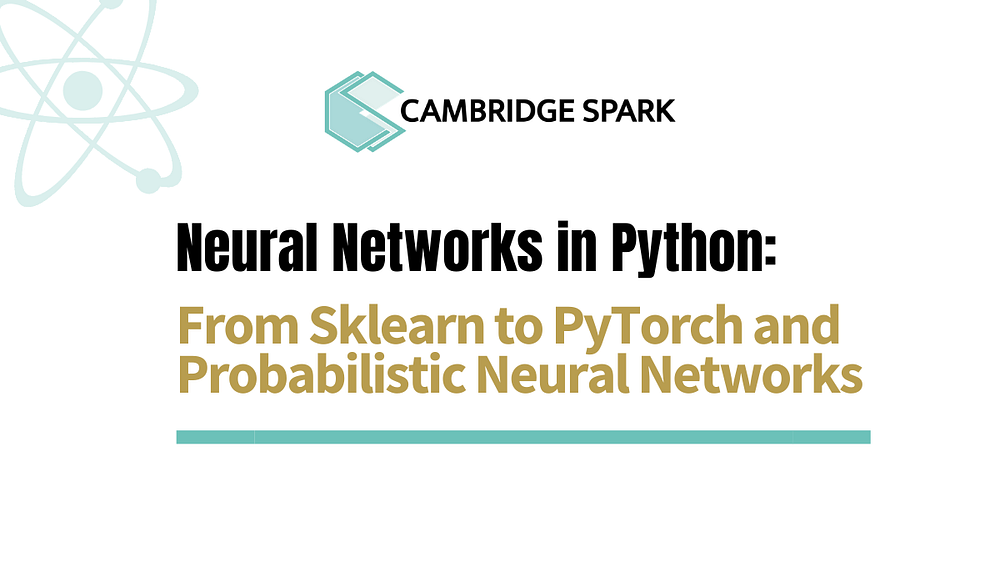# Neural Networks in Python: From Sklearn to PyTorch and Probabilistic Neural Networks

Source: Deep Learning on MediumThis tutorial covers different concepts related to neural networks with Sklearn and PyTorch. Neural networks have gained lots of attention in machine learning (ML) in the past decade with the development of deeper network architectures (known as deep learning). These models have even surpassed human capabilities in different vision and natural language processing datasets. For example, a neural network trained on the well-known ImageNet object recognition database tells the difference between different breeds of dog with an error rate of just 4.58%. For comparison, the typical human gets around 5%. Read more about this here

In this tutorial, we will first see how easy it is to train multilayer perceptrons in Sklearn with the well-known handwritten dataset MNIST. Things will then get a bit more advanced with PyTorch. We will first train a network with four layers (deeper than the one we will use with Sklearn) to learn with the same dataset and then see a little bit on Bayesian (probabilistic) neural networks. This tutorial assumes some basic knowledge of python and neural networks.

# What exactly are scikit-learn and PyTorch?

• Scikit-learn is a free software machine learning library for Python which makes unbelievably easy to train traditional ML models such as Support Vector Machines or Multilayer Perceptrons.
• PyTorch is an open-source machine learning library based on Torch, used for coding deep learning algorithms and primarily developed by Facebook’s artificial intelligence research group.

# …and why should I care about Pytorch and Probabilistic Neural Networks?

1. Many people prefer PyTorch to TensorFlow. This is mainly because PyTorch allows for dynamic computational graphs (meaning that you can change the network architecture during running time, which is quite useful for certain neural network architectures) and it’s very easy to learn (building ML models is actually very intuitive, as we will see).
2. ML needs to account for uncertainty! Have you heard of probabilistic programming? It’s a programming paradigm in which you can easily specify probabilistic models and perform inference on them. These languages greatly simplify the task of creating systems that handle uncertainty. For example, Pyro (from Uber AI Labs) enables flexible and expressive deep probabilistic modelling, unifying the best of modern deep learning and Bayesian modelling. And while we won’t touch on probabilistic programming in this tutorial, you may want to know why probabilistic approaches are so needed in ML and why these languages are growing so quickly. The network we are going to build doesn’t use probabilistic programming languages, but it’s still probabilistic!

Anyway… let’s get to it, shall we?

Let’s start with Sklearn and then we will move to PyTorch and finally include some notions of PNNs into the equation…

# Multilayer Perceptron in Sklearn to classify handwritten digits

The dataset we are going to use (MNIST) is still one of the most used benchmarks in computer vision tasks, where one needs to go from an image of a handwritten digit to the digit itself (0, 1, 2…). This could be done with a Convolutional Neural Network, which is the state-of-the-art method for discovering spatial patterns. However, to simplify this tutorial what we will do is to unroll/flatten the image into a vector (images are 28×28 pixels, which will result in a vector of size 784, where each element represents a pixel) and use a fully connected neural network.

What we are aiming to do is to build a mathematical function that can predict the characteristic of interest (digit) based on the pixels. This is where neural networks come in handy, as they are mathematical functions that are universal approximators (can approximate any function given enough degrees of freedom). Neural networks implement linear functions. However, they can also include nonlinear transformations known as activation units (for example a logistic function), which allows them to provide non-linear decision regions!

Let’s see how easy it would be to do so in Sklearn… We will build both a simple linear perceptron and a multilayer perceptron with the default activation functions in Sklearn, which are the so-called ReLU. When you run the code don’t forget to compare the accuracy of both models and play around with the hyperparameters and network architecture!